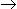# Chemical Engineering - Chemical Reaction Engineering - Discussion

4.

The fractional volume change of the system for the isothermal gas phase reaction, A3B , between no conversion and complete conversion is

 [A]. 0.5 [B]. 1 [C]. 2 [D]. 3

Explanation:

No answer description available for this question.

 K.Srijanya said: (Aug 16, 2013) The answer is 2, A---->3B HERE A REACTANT(input) B PRODUCT(output) SO WE HAVE A FORMULA (output-input)/input By this we can get like (3-1)/1 = 2.

 Surendra.D said: (Sep 14, 2013) The answer is 2: Because one mole of A gives 3 moles of B. And here don't give any conversion fractions. So we are simply use the formula product moles-reactant moles. After divide with reactant moles.

 Sudip Chowra said: (Sep 27, 2014) Use volume expansion factor. [(volm at 100% conversion-volm at 0% conversion)/volm at 100% conversion]

 Jaydeep Jani said: (Sep 28, 2014) V = V0(1+(volm at 100% conversion-volm at 0% conversion)/volm at 100% conversion)Xa). Put Xa=1. Then, V = 3V0. So fractional value changes from 1 to 3.

 Gani said: (Dec 14, 2014) This is a gas phase reaction density is not constant. So e = [Total no of moles of products-Total no of moles of reactants]\Total no of moles of reactants.